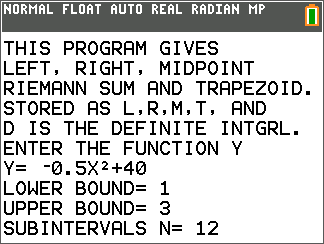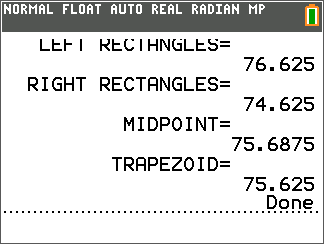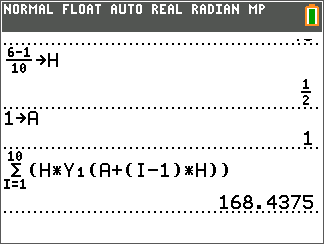# Activities

••• ##### Subject Area

• Math: Calculus: Antiderivatives and Slope Fields

• ##### Author9-12

45 Minutes

• ##### Device
• TI-84 Plus
• TI-84 Plus Silver Edition
•TI-84 Plus C Silver Edition
•TI-84 Plus CE
• ##### Software

TI Connect™ CE

## Approximating the Area Under a Curve#### Activity Overview

In this activity, students will explore approximating the area under a curve using left endpoint, right endpoint, and midpoint Riemann sums.

#### Key Steps

•Students will be presented with the function and be asked to calculate three different approximations for the area under its curve.

•Student will be asked to calculate the value of the definite integral and compare it to the four approximations of the area found earlier.

•Student will use the sigma notation to evaluate the left Riemann sum.